Alg 1 M1 U2
starstarstarstarstarstarstarstarstarstar
by Gina Spencer
| 36 Questions
Note from the author:
Algebra Assessment
Use desmos.com to plot the data below. Then find the least squares line and the correlation coefficient.
For your answers, round numbers to the nearest thousandth. Answer questions 1-5 using the results from Desmos: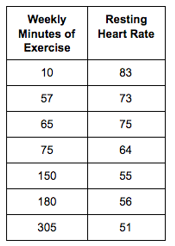1
1
Least Squares Line (line of best fit, in slope-intercept form), do not include spaces, round to 3 decimals for each term:
2
1
Value of Correlation Coefficient (r=__________, round to 3 decimals):
3
2
Circle the words below that describe the correlation between resting
heart rate and weekly minutes of exercise. (Select all that apply)
Weak
Moderate
Strong
Perfect Correlation
No Correlation
Positive
Negative
4
1
What is the slope of your least squares line? (Round to the nearest thousandth)
5
1
Interpret the results of the data given the context of the problem
6
2
Which of the following is the correct interpretation for the y-intercept of your least squares line?
It is the estimated value of weekly minutes of exercise when resting heart rate = 0.
It is the estimated value of resting heart rate when weekly minutes of exercise = 0.
It is the rate of change for resting heart rate.
None of the above.
7
2
Does the y-intercept of your least squares line make sense in the context of the problem? Explain.
The graph shows a store’s daily ice cream sales (in dollars) and maximum temperatures for that day (in degrees Fahrenheit). The line of best fit is given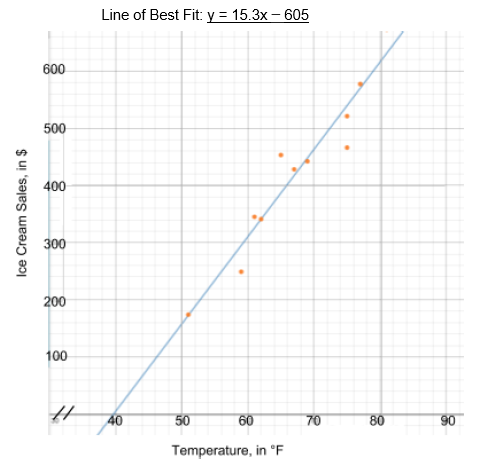8
2
Use the equation of the line of best fit (not the graph alone) to predict daily ice cream sales (in dollars) on a day when the maximum temperature is 77 degrees Fahrenheit. Round your answer to the nearest thousandth.
9
1
Assume the actual daily ice cream sales were \$548.50 on the day when the maximum temperature was 77 degrees Fahrenheit. Compare this to your previous answer from Question 5 and calculate the residual.
10
2
Use either the graph or the equation of the line of best fit to predict maximum temperature on a day when ice cream sales were \$460. (For full credit: If you use the graph, mark it to show how you found your answer; if you use the equation, show your work below the graph using a text box.)
11
2
Using the information from question #10 answer the following: Does the y-intercept of your least squares line make sense in the context of the problem? Explain.
Order these graphs from strongest correlation to weakest correlation represented.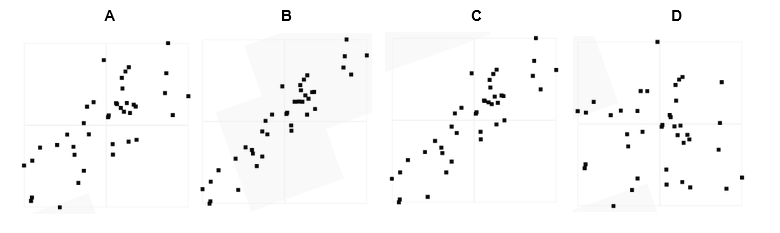12
1
Very Strong Correlation
A
B
C
D
13
1
Strong Correlation
A
B
C
D
14
1
Weak Correlation
A
B
C
D
15
1
Weakest Correlation
A
B
C
D
Use the Graphs below to answer the questions: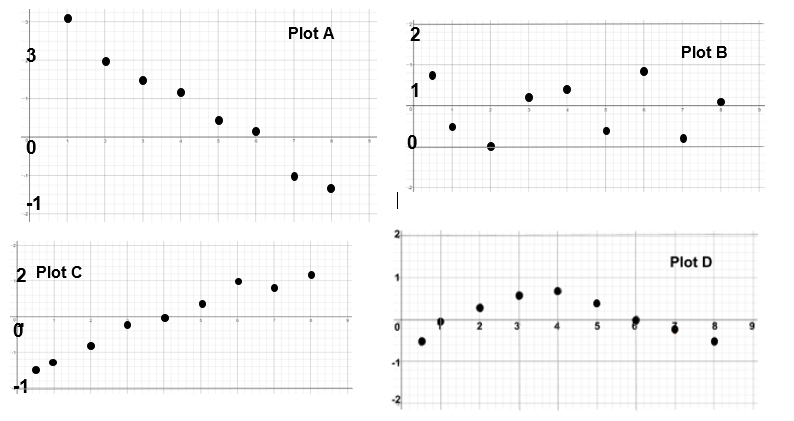16
2
Assume you entered data about x and y into Desmos and found a least squares regression line (a line of best fit). You then check the residuals resulting from that line. Which of the residual plot below would best indicate that the line is a good fit for the data?
Plot A because it shows a linear pattern.
Plot B because it shows a random distribution of residuals.
Plot C because there are about the same number of positive and negative residuals.
Plot D because all of the residuals are close to 0.
Natalie and Cam are playing “guess that point" with the data and line of best fit shown below.17
2
Natalie says: I’m thinking of a point that has a residual of 2. Which point is Natalie thinking of?
Point A
Point B
Point C
Point D
Point E
Point F
Use the graph below to answer the following question:18
1
Which of the following equations most closely matches the line of best fit on the scatter plot shown above?
y = 2x + 4
y=1/2 x + 4
y = 4x + 2
y = 1/4 x + 2
19
1
Before you start working, you have \$20. When you start working, you make \$15 for every 1 hour you work.
Which of the following equations represents this scenario?
y = 20x + 15, where y = number of hours worked and x = amount of money in \$
y = 20x + 15, where y = amount of money in \$ and x = number of hours worked
y = 15x + 20, where y = number of hours worked and x = amount of money in \$
y = 15x + 20, where y = amount of money in \$ and x = number of hours worked
20
1
If the correlation coefficient is − 0.897, which of the following statements must be true? Select all that apply
There is a weak correlation between the two sets of data.
There is a strong correlation between the two sets of data.
The first set of data causes the second set of data.
As the horizontal values increase the vertical values increase.
As the horizontal values increase the vertical values decrease.
For questions 21 - 25, match each graph to the statements below. Use each graph one time only.21
1
This graph shows a negative linear correlation.
Graph A
Graph B
Graph C
Graph D
Graph E
22
1
This graph shows a nonlinear exponential relationship.
Graph A
Graph B
Graph C
Graph D
Graph E
23
1
This graph shows a positive linear correlation.
Graph A
Graph B
Graph C
Graph D
Graph E
24
1
This graph shows a nonlinear quadratic relationship.
Graph A
Graph B
Graph C
Graph D
Graph E
25
1
This graph shows no correlation.
Graph A
Graph B
Graph C
Graph D
Graph E
For Questions 26 – 29, match each graph to the statement that makes the most sense. Use each graph one time only. All axes labels have been deleted from the graphs.26
1
Ice cream sales increase steadily as the temperature increases.
Graph A
Graph B
Graph C
Graph D
27
1
As the temperature increases the sales of hot chocolate decrease.
Graph A
Graph B
Graph C
Graph D
28
1
The number of DVD players sold took a while to increase, then increased each year and finally has reached a level period with little change in the number sold.
Graph A
Graph B
Graph C
Graph D
29
1
There doesn’t seem to be any pattern in the cost of toys produced versus toy sales in those countries.
Graph A
Graph B
Graph C
Graph D
Use the graph to answer problems 30-33.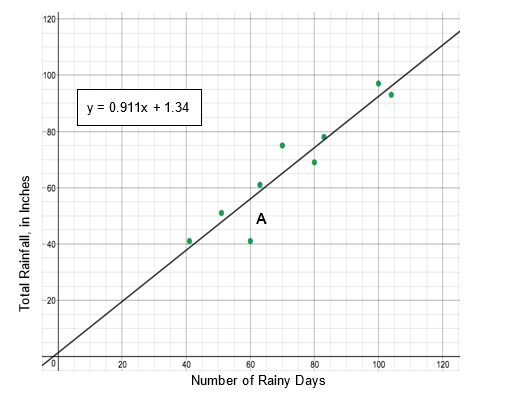30
1
Which of the following interpretations is correct?
A slope of 1.34 means that a 1.34 inch increase in total rainfall is predicted for every 1 additional rain day.
A slope of 0.911 means that a 0.911 (day) increase in number of rain days is predicted for every 1 additional inch of total rainfall.
A slope of 1.34 means that a 1.34 (day) increase in number of rain days is predicted for every 1 additional inch of total rainfall.
A slope of 0.911 means that a 0.911 inch increase in total rainfall is predicted for every 1 additional rain day.
31
1
If you added the point (85, 103) to the data set shown in this scatterplot, how would this influence the line of best fit?
The line of best fit would remain the same
Without also using technology, it cannot be determined how the line of best fit would change
The slope of the line of best fit would increase
The slope of the line of best fit would decrease
32
1
If there were 120 rain days, based on the line of best fit, what would you expect total rainfall to be?
100
110
It cannot be determined
90
33
1
What is the value of the residual for Point A?
60
41
-15
15
It residual cannot be determined
34
1
Which of the following are true? (Select all that apply)
Strong correlations can be positive or negative.
A correlation coefficient of 0.72 suggest that as x increases, y decreases.
A perfect correlation (r= 1 or r=-1) suggests that changes in x cause change in y.
A correlation coefficient of 0.17 suggests a stronger correlation than a correlation coefficient of -0.27 because it is positive.
A correlation of 0 indicates no relationship between the two variables.
Bonus Questions (1 point each)
On vacation, you plan to rent an electric scooter for a flat fee of \$20 plus an hourly fee of \$8.
35
0
Write an equation to represent the situation.
36
0
How much would you pay to use the scooter for 4 hours?